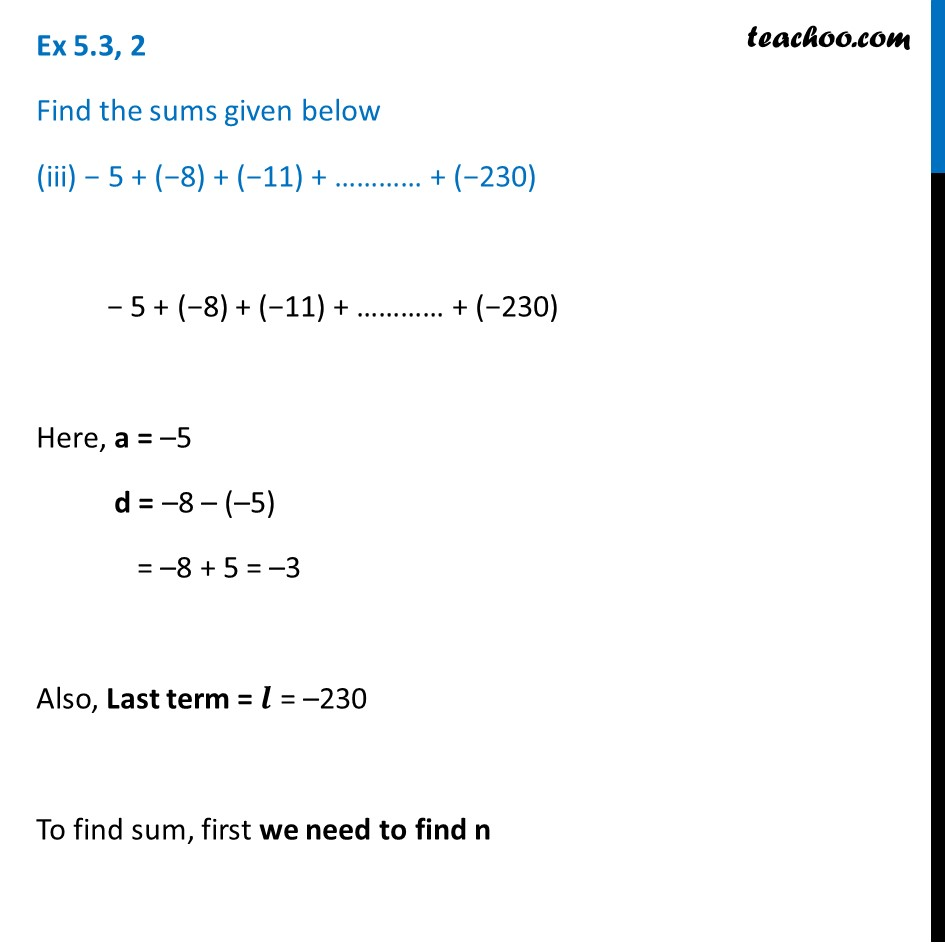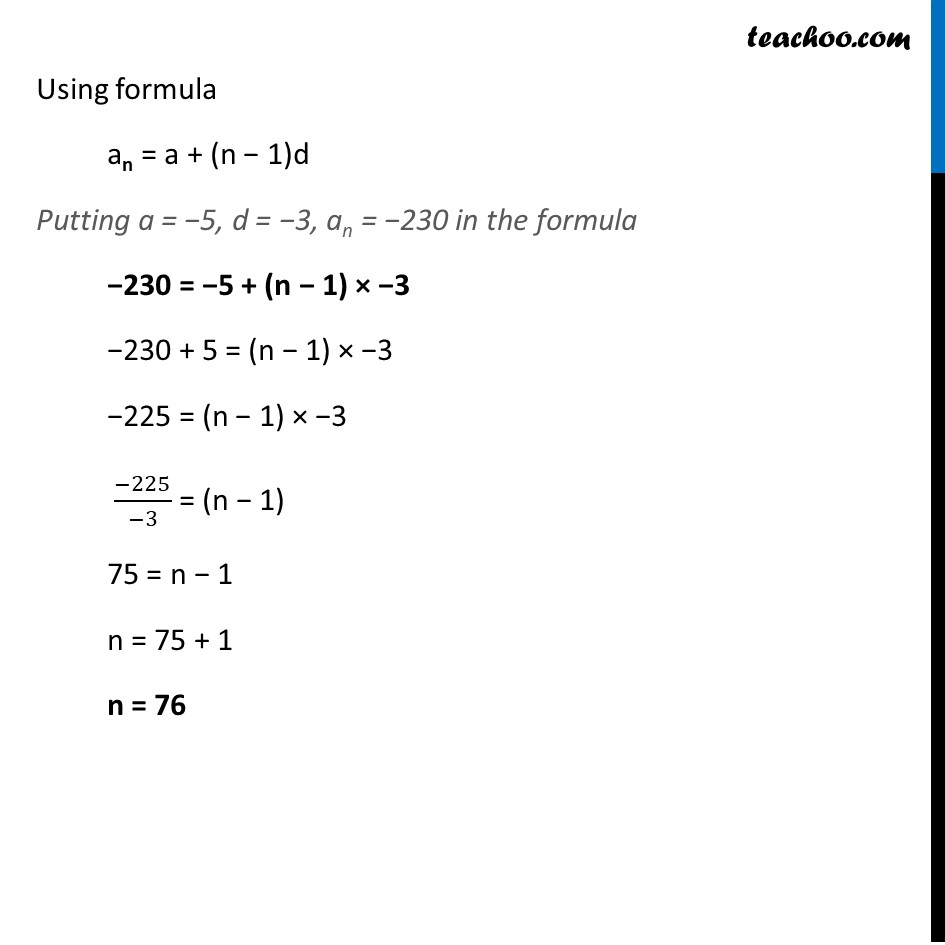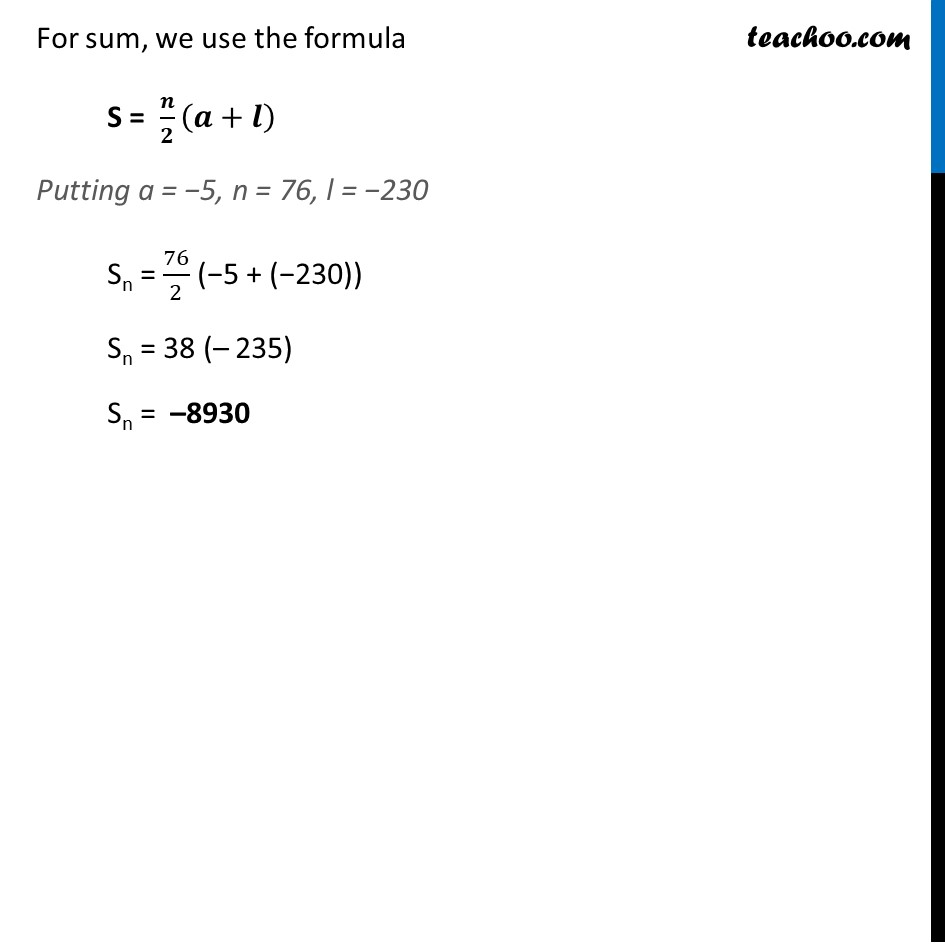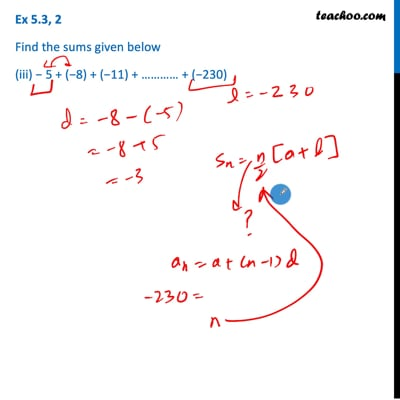Ex 5.3

Chapter 5 Class 10 Arithmetic Progressions (Term 2)
Serial order wiseThis video is only available for Teachoo black users

### Transcript

Ex 5.3, 2 Find the sums given below (iii) − 5 + (−8) + (−11) + ………… + (−230) − 5 + (−8) + (−11) + ………… + (−230) Here, a = –5 d = –8 – (–5) = –8 + 5 = –3 Also, Last term = 𝒍 = –230 To find sum, first we need to find n Using formula an = a + (n − 1)d Putting a = −5, d = −3, an = −230 in the formula −230 = −5 + (n − 1) × −3 −230 + 5 = (n − 1) × −3 −225 = (n − 1) × −3 (−225)/(−3) = (n − 1) 75 = n − 1 n = 75 + 1 n = 76 For sum, we use the formula S = 𝒏/𝟐 (𝒂+𝒍) Putting a = −5, n = 76, l = −230 Sn = 76/2 (−5 + (−230)) Sn = 38 (– 235) Sn = –8930